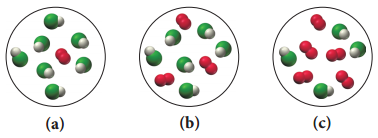# Problem: Consider the reaction:4 HCl(g) + O2(g) → 2 H2O(g) + 2 Cl2(g)Each molecular diagrams represents an initial mixture of the reactants.Which mixture produces the greatest amount of products?

###### FREE Expert Solution

a.

= 3.5 mol H2O

= 0.5 mol H2O → limiting

b.

= 2.5 mol H2O

= 1.5 mol H2O → limiting

97% (192 ratings)###### Problem Details
Consider the reaction:

4 HCl(g) + O2(g) → 2 H2O(g) + 2 Cl2(g)

Each molecular diagrams represents an initial mixture of the reactants.Which mixture produces the greatest amount of products?

Frequently Asked Questions

What scientific concept do you need to know in order to solve this problem?

Our tutors have indicated that to solve this problem you will need to apply the Limiting Reagent concept. You can view video lessons to learn Limiting Reagent. Or if you need more Limiting Reagent practice, you can also practice Limiting Reagent practice problems.